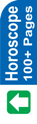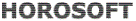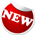Astrology Software
Indian AstrologyZODIACAL & PLANETARY PRODUCTS Before we study the uses of dots in different Ashtakvargas, there are two more factors to be studied. These are Zodiacal Product and Planetary Product . Zodiacal Products These can be found out by the following steps : 1. Find out the no. of dots in each Ashtakvarg (after 2nd reduction, i.e. Ekadhipati Shodan, 2. Multiply each figure by the zodiacal factors for each sign. These are constant for all horoscopes and are given below : Signs : 1 2 3 4 5 6 7 8 9 10 11 12 Zodiacal 7 10 8 4 10 5 7 8 9 5 11 12 Factors : For example, let us find out Zodiacal Product in case of Sun Ashtakvarga for our sample horoscope. Already we have worked out the no. of dots after Ekadhipati Shodan . These are : Signs : 1 2 3 4 5 6 7 8 9 10 11 12 Sun : 0 2 0 2 5 3 0 0 3 0 1 2 Multiplying by 7 10 8 4 10 5 7 8 9 5 11 12 Zodiacal factors Result : 0 20 0 8 50 15 0 0 27 0 11 24 The total of these comes to 146. This is the Zodiacal Product for Sun. This way, the zodiacal products for all planets can be found out. These have been worked out and summarised in a tabular form below. Calculation of Zodiacal Products for all planets SN Sun Moon Mars Merc Jup Venus Sat 1 0x7 = 0 1x7 = 7 0x7 = 0 0x7 = 0 0x7 = 0 0x7 = 0 0x7 = 0 2 2x10=20 0x10=0 0x10=0 0x10=0 0x10=0 0x10=0 0x10=0 3 0x8 = 0 0x8 = 0 0x8 = 0 0x8 = 0 1x8 = 8 0x8 = 0 1x8 = 8 4 2x4 = 8 5x4=20 0x4=0 0x4=0 3x4=12 1x4=4 2x4=8 5 5x10=50 3x10=30 3x10=30 4x10=40 2x10=20 1x10=10 2x10=20 6 3x5=15 0x5=0 4x5=20 2x5=10 1x5=5 0x5=0 1x5=5 7 0x7= 0 0x7= 0 1x7= 7 2x7=14 0x7=0 1x7=7 2x7=14 8 0x8 = 0 0x8 = 0 0x8 = 0 0x8 = 0 3x8=24 1x8=8 0x8=0 9 2x9=18 0x9=0 1x9=9 0x9=0 0x9=0 1x9=9 0x9=0 10 0x5 =0 1x5 =5 0x5 =0 0x5 =0 0x5 =0 1x5 =5 1x5 =5 11 1x11=11 0x11=0 0x11=0 1x11=11 3x11=33 0x11=0 0x11=0 12 2x12=24 4x12=48 1x12=12 0x12=0 0x12=0 0x12=0 3x12=36 * 146 110 78 75 102 43 96 * Total Rasi Pind for each planet Planetary Products These can be found out by the following steps : 1. Find out the no. of dots in the signs where different planets are posited in each Ashtakvarg (after 2nd reduction, i.e. Ekadhipati Shodan), 2. Multiply each figure by the planetary factors for each sign. These are constant for all horoscopes and are given below : Sun = 5 Mon= 5 Mar= 8 Mer = 5 Jup = 10 Ven = 7 Sat = 11 For example, let us find out planetary product in case of Sun Ashtakvarga for our sample horoscope. Already we have worked out the no. of dots after Ekadhipati Shodan. We also find from the sample horoscope that Sun is in 8, Mon in 7, Mars in 8, Mer in 8, Jup in 7, Ven in 8 and Sat in 11. This means signs involved are 8, 7, 8,7,8,8, and 11, i.e. 7, 8, and 11. The dots in sign 8 in Sun Ashtakvarga is 0, in sign 7 also 0, and in sign 11, it is 1. Now in each Ashtakvarga find out the number(s) in the sign (s) in case of each planet and multiply the same by Planetary factors as given below : No. of dots (after Ekadhipati Shodan) in each sign in Sun Ashtakvarga are (as already worked out) : Signs : 1 2 3 4 5 6 7 8 9 10 11 12 Sun : 0 2 0 2 5 3 0 0 3 0 1 2 As Sun is in 8, multiply the no. of dots in sign 8 in Sun Ashtakvarga, i.e. 0 by the planetary factor for Sun, which is 5. The result will be 0 ( 0 x 5). As Moon is in 7, multiply the no. of dots in sign 7 in Sun Ashtakvarga, i.e. 0 by the planetary factory for Moon, which is also 5. The results will be 0 (0 x 5). Similarly these have been worked out for all Ashtakvargas and shown in the following table. Sun Mon Mar Mer Jup Ven Sat Sun in 8 0x5=0 0x5=0 0x5=0 0x5=0 3x5=15 1x5=5 0x5=0 Mon in 7 0x5=0 0x5=0 1x5=5 2x5=10 0x5=0 1x5=5 2x5=10 Mar in 8 0x8=0 0x8=0 0x8=0 0x8=0 3x8=24 1x8=8 0x8=0 Mer in 8 0x5=0 0x5=0 0x5=0 0x5=0 3x5=15 1x5=5 0x5=0 Jup in 7 0x10=0 0x10=0 1x10=10 2x10=20 0x10=0 1x10=10 2x10=20 Ven in 8 0x7=0 0x7 0x7=0 0x7=0 3x7=21 1x7=7 0x7=0 Sat in 11 1x5=5 0x5=0 0x5=0 1x5=5 3x5=15 0x5=0 0x5=0 Total 5 0 15 35 90 40 30 Thus, Sun Mon Mar Mer Jup Ven Sat Zodiacal Products 146 110 78 75 102 43 96 Planetary Products 5 0 15 35 90 40 30 Sodya* Products 151 110 93 110 192 83 126 *Sodya meaning for which shodhan has been done, i.e. reduction. Some write it as pure or real. After calculating these products, let us now find out how to use these products. Examples (relating to sample horoscope) 1. Sodya Pinda of Sun : 151 No. of dots in the IXth (before reduction) from Sun in Sun Ashtakvarga (Chart No. 9) = 5 Multiply these two figures, i.e. 151 x 5 = 755 Divide this figure by 27, i.e. = 755/27 = 27 26/27. This means remainder is 26. Now count the 26th star from Aswini. This comes to Uttar Bhadrapad. Whenever Saturn passes over this star, there will be some trouble to father of the native. 2. Sodya Pinda of Moon = 110 No. of dots in the IVth (before reduction) from Moon in the Moon Ashtakvarga = 5 Multiply these two figures, i.e. 110 x 5 = 550 Divide this by 27, i.e. 550/27 = 20 10/27. This means remainder is 10. Now count the 10th star from Aswini. This comes to Magha. Whenever Saturn passes over this star, there will be some trouble to mother of the native. 3. Sodya Pinda of Mars = 93 No. of dots in the IIIrd (before reduction) from Mars in the Mars Ashtakvarga = 2 Multiply these two figures, i.e. 93 x 2 = 186 Divide this by 27, i.e. 186/27 = 6 24/27. This means remainder is 24. Now count the 24th star from Aswini. This comes to Shatbisha. Whenever Saturn passes over this star, there will be some trouble to brother of the native. 4 Sodya Pinda of Mercury = 110 No. of dots in the Xth (before reduction) from Mercury in the Mercury Ashtakvarga = 6 Multiply these two figures, i.e. 110 x 6 = 660 Divide this by 27, i.e. 660/27 = 24 12/27. This means remainder is 12. Now count the 12th star from Aswini. This comes to Uttar Phalguni. Whenever Saturn passes over this star, there will be losses in the business/profession of the native. . 5. Sodya Pinda of Jupiter = 192 No. of dots in the Vth (before reduction) from Jupiter in the Jupiter Ashtakvarga = 5 Multiply these two figures, i.e. 192 x 5 = 960 Divide this by 27, i.e. 960/27 = 35 15/27. This means remainder is 15. Now count the 15th star from Aswini. This comes to Swati. Whenever Jupiter passes over this star, there will be birth of a child to the native. 6. Sodya Pinda of Venus= 83 No. of dots in the VIIth (before reduction) from Venus in the Venus Ashtakvarga = 4. Multiply these two figures, i.e. 83 x 4 = 332 Divide this by 27, i.e. 332/27 = 12 8/27. This means remainder is 8. Now count the 8th star from Aswini. This comes to Pushya. Whenever Jupiter passes over this star, marriage of the native may take place. 7. Sodya Pinda of Venus= 83 No. of dots in the VIIth (before reduction) from Jupiter in the Venus Ashtakvarga = 3 Multiply these two figures, i.e. 83 x 3 = 249 Divide this by 27, i.e. 249/27 = 9 6/27. This means remainder is 6. Now count the 6th star from Aswini. This comes to Ardra. Whenever Saturn passes over this star, there will be trouble to husband of the female native. 8. 8. Sodya Pinda of Saturn = 126 No. of dots in the VIIIth (before reduction) from Saturn in the Saturn Ashtakvarga = 5. Multiply these two figures, i.e. 126 x 5 = 630 Divide this by 27, i.e. 630/27 = 23 9/27. This means remainder is 9. Now count the 9th star from Aswini. This comes to Ashlesha. Whenever Saturn passes over this star, trouble to or even death of the native may occur. Thus, keeping in mind the basic principles of Astrology, these products can be used to find out several answers. Always remember to balance the picture in your mind. Try to tally the time being suggested by transit with the Vimshottari Dasha.Astrowealth Astrology Software Software for Website Astrology Reports Free Services Regional Language Important Link